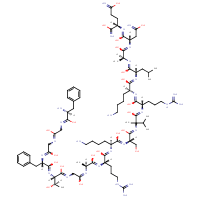Structure InformationCompound Identification

SMILES

CC(C)C[C@H](NC(=O)[C@H](CCCCN)NC(=O)[C@H](CCCNC(N)=N)NC(=O)C(C)(NC(=O)[C@H](CO)NC(=O)[C@H](CCCCN)NC(=O)[C@H](CCCNC(N)=N)NC(=O)[C@H](C)NC(=O)CNC(=O)[C@@H](NC(=O)[C@H](CC1=CC=CC=C1)NC(=O)CNC(=O)CNC(=O)[C@@H](N)CC1=CC=CC=C1)[C@@H](C)O)C(C)C)C(=O)N[C@@H](C)C(=O)N[C@@H](CC(N)=O)C(=O)N[C@@H](CCC(N)=O)C(N)=O

InChIKey

InChIKey=CWWCCESEUORKQB-AGNWCVJKSA-N

Formula

C82H136N28O21

Mass

1850.165

### Export to:

Taxonomic Classification

Taxonomy Tree

Kingdom

Organic compounds

Superclass

Organic Polymers

Class

Polypeptides

Subclass

Not available

Intermediate Tree Nodes

Not available

Direct Parent

Polypeptides

Alternative Parents

Molecular Framework

Aromatic homomonocyclic compounds

Substituents

Polypeptide - Alpha peptide - Phenylalanine or derivatives - Arginine or derivatives - Glutamine or derivatives - Leucine or derivatives - Asparagine or derivatives - N-acyl-alpha amino acid or derivatives - Alpha-amino acid amide - Serine or derivatives - Alanine or derivatives - N-substituted-alpha-amino acid - Alpha-amino acid or derivatives - Amphetamine or derivatives - Aralkylamine - Fatty amide - N-acyl-amine - Fatty acyl - Monocyclic benzene moiety - Benzenoid - Amino acid or derivatives - Guanidine - Carboxamide group - Primary carboxylic acid amide - Secondary alcohol - Secondary carboxylic acid amide - Carboxylic acid derivative - Carboximidamide - Alcohol - Imine - Primary aliphatic amine - Organonitrogen compound - Organooxygen compound - Carbonyl group - Primary alcohol - Primary amine - Hydrocarbon derivative - Amine - Organic oxide - Organic oxygen compound - Organic nitrogen compound - Aromatic homomonocyclic compound

Description

This compound belongs to the class of organic compounds known as polypeptides. These are peptides containing ten or more amino acid residues.

External Descriptors

Not available